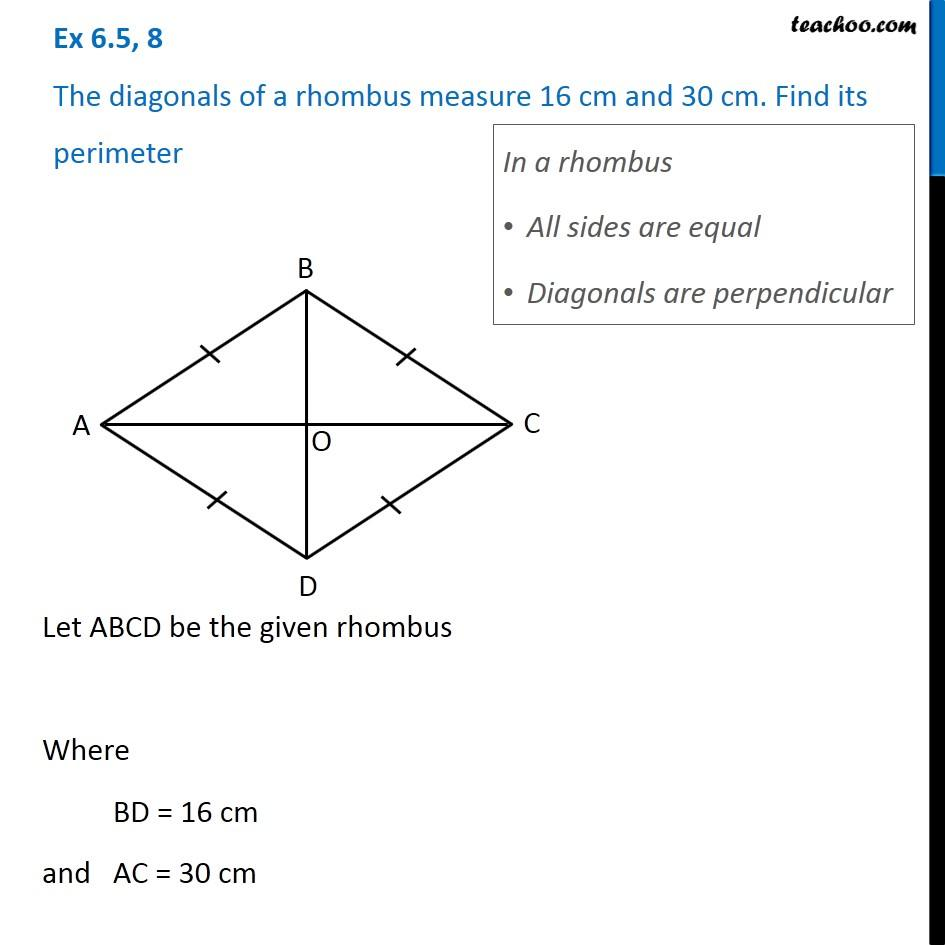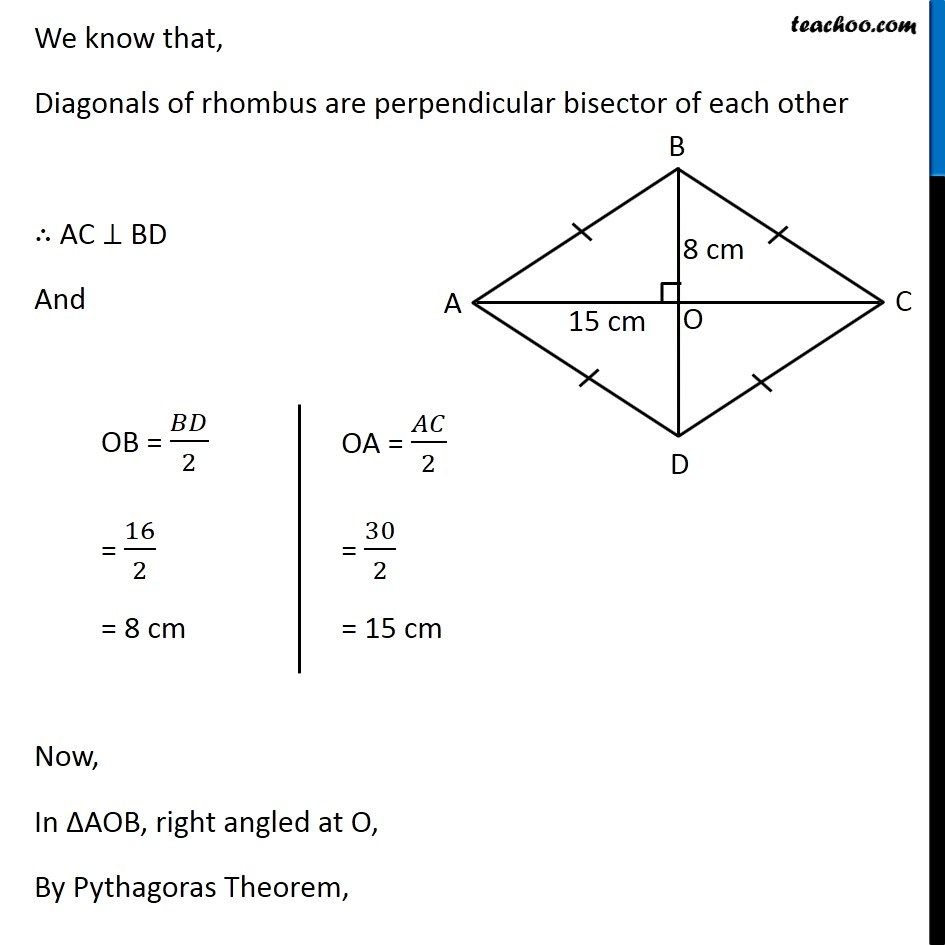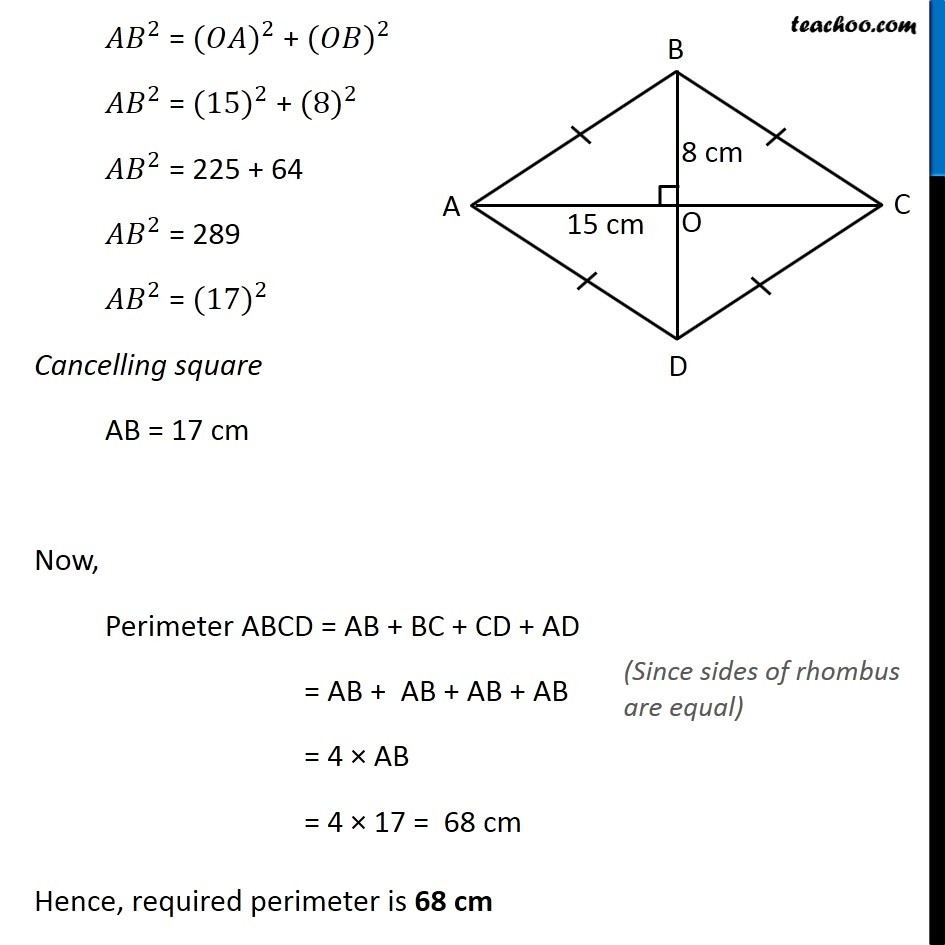Ex 6.5

Chapter 6 Class 7 Triangle and its Properties
Serial order wiseLearn in your speed, with individual attention - Teachoo Maths 1-on-1 Class

### Transcript

Ex 6.5, 8 The diagonals of a rhombus measure 16 cm and 30 cm. Find its perimeter In a rhombus All sides are equal Diagonals are perpendicular Let ABCD be the given rhombus Where BD = 16 cm and AC = 30 cm We know that, Diagonals of rhombus are perpendicular bisector of each other ∴ AC ⊥ BD And OB = 𝐵𝐷/2 = 16/2 = 8 cm OA = 𝐴𝐶/2 = 30/2 = 15 cm Now, In ∆AOB, right angled at O, By Pythagoras Theorem, 〖𝐴𝐵〗^2 = 〖(𝑂𝐴)〗^2 + 〖(𝑂𝐵)〗^2 〖𝐴𝐵〗^2 = 〖(15)〗^2 + 〖(8)〗^2 〖𝐴𝐵〗^2 = 225 + 64 〖𝐴𝐵〗^2 = 289 〖𝐴𝐵〗^2 = 〖(17)〗^2 Cancelling square AB = 17 cm Now, Perimeter ABCD = AB + BC + CD + AD = AB + AB + AB + AB = 4 × AB = 4 × 17 = 68 cm Hence, required perimeter is 68 cm (Since sides of rhombus are equal)